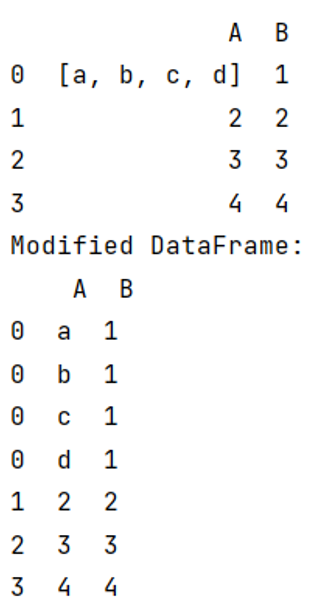# Split (explode) pandas DataFrame string entry to separate rows

Given a DataFrame, we have to split (explode) string entry to separate rows. By Pranit Sharma Last updated : September 20, 2023

Rows in pandas are the different cell (column) values that are aligned horizontally and also provide uniformity. Each row can have the same or different value. Rows are generally marked with the index number but in pandas, we can also assign index names according to the needs. In pandas, we can create, read, update and delete a column or row value.

## Problem statement

Given a DataFrame, we have to split (explode) string entry to separate rows.

## Splitting (exploding) pandas DataFrame string entry to separate rows

For this purpose, we will use pandas.DataFrame.explode() method. This method transforms each element of a list-like to a row, replicating index values.

The following is the syntax of the explode() method:

```DataFrame.explode(column, ignore_index=False)
```
Note

To work with pandas, we need to import pandas package first, below is the syntax:

```import pandas as pd
```

Let us understand with the help of an example:

## Python program to split (explode) pandas DataFrame string entry to separate rows

```# Importing Pandas package as pd
import pandas as pd

# Creating a dictionary
d = {'A':[['a','b','c','d'],2,3,4],'B':[1,2,3,4]}

# Creating DataFrame
df = pd.DataFrame(d)

# Display original DataFrame
print(df)

# Expanding a particular column
result = df.explode('A')

# Display Result
print("Modified DataFrame:\n",result)
```

### Output

The output of the above program is: